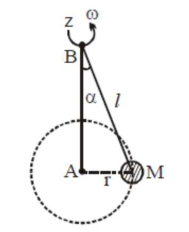# A mass M hangs on a massless rod of length l which rotates at a constant angular frequency.Question:

A mass $M$ hangs on a massless rod of length $l$ which rotates at a constant angular frequency. The mass $\mathrm{M}$ moves with steady speed in a circular path of constant radius. Assume that the system is in steady circular motion with constant angular velocity $\omega$. The angular momentum of $\mathrm{M}$ about point $\mathrm{A}$ is $\mathrm{L}_{\mathrm{A}}$ which lies in the positive $z$ direction and the angular momentum of $\mathrm{M}$ about $\mathrm{B}$ is $\mathrm{L}_{\mathrm{B}}$. The correct statement for this system is :1. $\mathrm{L}_{\mathrm{A}}$ and $\mathrm{L}_{\mathrm{B}}$ are both constant in magnitude and direction

2. $\mathrm{L}_{\mathrm{B}}$ is constant in direction with varying magnitude

3. $\mathrm{L}_{\mathrm{B}}$ is constant, both in magnitude and direction

4. $\mathrm{L}_{\mathrm{A}}$ is constant, both in magnitude and direction

Correct Option: , 4

Solution:

(4)

We know, $\overrightarrow{\mathrm{L}}=\mathrm{m}(\overrightarrow{\mathrm{r}} \times \overrightarrow{\mathrm{v}})$\

Now with respect to A, we always get direction

of $\overrightarrow{\mathrm{L}}$ along tve z-axis and also constant magnitude as mvr. But with respect

to $\mathrm{B}$, we get constant magnitude but continuously changing direction.